# Simulation of the sound propagation inside a wind instrument by means of the Finite-Difference Time-Domain Method

J. Redondoa, R. Picoa and B. Roigb

a Grupo de Dispositivos y Sistemas Acústicos y Ópticos, DISAO. Escuela Politécnica Superior de Gandía Carretera Nazaret Oliva S/N, Grao de Gandia, Spain

b Departamento de Matemática Aplicada. Escuela Politécnica Superior de Gandia. Carretera Nazaret Oliva S/N, Grao de Gandia, Spain

RESUMEN: En el presente trabajo se estudia la evolución temporal de la propagación de las ondas sonoras en el interior de un instrumento de viento mediante el método de diferencias finitas en el dominio temporal (FDTD). El modelo de instrumento adoptado se compone de un resonador con simetría axial (cuerpo del instrumento) excitado por medio de pulsos periódicos de aire. Para simular el mecanismo de excitación del instrumento se emplean fuentes transparentes en la entrada del resonador. La técnica de cálculo empleada en el método de diferencias finitas reduce las oscilaciones espureas ya que utiliza dos mallas al tresbolillo. Esta técnica ha sido utilizada en las últimas décadas en otros ámbitos de la Acústica, tales como la Acústica de Salas  o la Acústica Ambiental . La simulación numérica del instrumento permite analizar la influencia de la geometría interna (agujeros, forma de la terminación) en la propagación sonora del instrumento, así como, la evolución temporal del régimen de oscilación.

ABSTRACT: In the present work the time evolution of the travelling sound waves inside a wind instrument is studied by means of a Finite-Difference Time Domain (FDTD) method. The adopted model for the instrument consists of a resonator with axial symmetry (body of the instrument) excited by periodic air pulses. In order to simulate the excitation mechanism transparent sources should be used at the input of the resonator.  The considered calculation technique reduces the spurious oscillations due to the use of staggered grids. This technique has been successfully applied in the last decades in several fields within Acoustics, such as Room Acoustics  or Environmental Acoustics . The numerical simulation of the instrument makes possible to analyze the influence of the internal geometry (orifice, termination shape, and so on) on the sound propagation and the time evolution of the oscillation regime.

## 1. INTRODUTION

In the field of woodwind acoustics, different numerical methods have been employed to simulate the behaviour of acoustical bores. Some of this works are applied in sound synthesis by physical modelling of woodwind, such as digital waveguide modelling (DWM)  and the multi convolution algorithm (MCA)  or the wave digital modelling (WDM) .  The approach is based on a one-dimensional model of wave propagation in the bore. In this work, the Finite-difference time-domain method (FDTD) is used to evaluate the acoustic response of a simplified instrument consisting of a cylindrical pipe excited at the entrance.

2. TIME DOMAIN SIMULATION USING FINITE DIFFERENCE

The finite-difference time-domain (FDTD) method was first introduced by Yee in 1966  in order to study the scattering of electromagnetic waves. Since that moment a counterpart for acoustics has been developed in several fields, mainly in room acoustics. In the case of musical instruments authors have mainly use different numeric techniques like Finite Element Methods (FEM) and Boundary Element Methods (BEM). In most of the cases frequency domain methods were considered. In last decade some extensions of those methods to the time domains has been used in order to analyze transient states.

### 2.1 Fundamentals

The first order acoustic equations in a homogeneous medium without losses can be written as follows,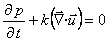(1)(2)

where p is the pressure field,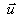is the vector particle velocity field,is the mass density of the medium and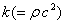is the compressibility of the medium.

In the case of axial symmetric sound fields, equations (1) and (2) can be written in cylindrical coordinates as follows,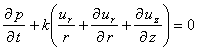(3)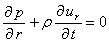(4)(5)

The spatial and time derivatives of any function can be approximated by central finite difference. For instance,(6)

where Dr is the spatial interval between considered points. Defining three staggered grids (see figure 1) and after some algebra equations (3)-(5) can be expressed as follows:(7)(8)(9)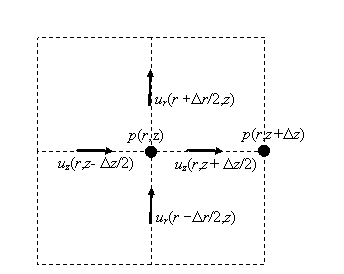Figure 1 – Spatial staggered grids considered So, the pressure, p, is evaluated in a discrete mesh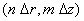at timeswhile the velocity, ur and uz, are evaluated in slightly displaced meshes (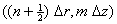andrespectively) at times(m, n and l are integers). This scheme is known as a time-spatial staggered grid that increases the accuracy.

Assuming a locally reactive boundary, the particle velocity on the boundary can be expressed as follows:(10)

whereis the vector normal to the boundary and Z is the acoustic impedance of the boundary. As an example we will express the boundary condition in the defined staggered grids defined above for a flat surface betweenand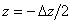,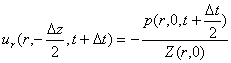(11)

2.2 Perfectly Matched Layers

Due to some cumulative errors using equation (10) for boundary conditions with impedance matching the acoustic impedance of the medium (r c), the absorption of the boundaries is limited. For practical reasons it is impossible to achieve level differences between reflected and incident waves bigger that 60 dB. Therefore a huge grid should be considered in order to separate in time the boundary spurious reflections from the significant reflections inside the integration area. The bigger the integration area is, the slower the calculations result. To avoid the need for huge integration areas a technique called “Perfectly Matched Layers” (PML) has been proposed [7-8]. This technique consists on defining a lossy medium in the zones near to the boundaries. This implies the inclusion of additional terms, including two attenuation factors (gr and gz) in the different spatial directions considered, i.e:(12)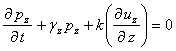(13)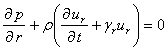(14)(15)

where the sound pressure p has been split into two additive components pr and pz. These components have no physical meaning and are defined only because of mathematical reasons.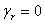inside the integration area                     (16), j = 1,…, m   at the j-th layer of the PML                 (17)

gz is defined in an analogous way. gmax is the maximum value of the attenuation coefficient.

2.3 Excitation (source inclusion)

Once defined the integration scheme is important to consider which of the possible excitation techniques should be used. Excitation techniques can be divided attending to the field that is excited, pressure or particle velocity, and attending to the way in which this excitation is included. In the second case one can simply impose a spatial excitation at time t = 0 or to include a time perturbation on at least one point of the grids. This means that the time evolution of one (or more) point is not governed by equations given above but from a discrete time function, i.e.:(18)

In order to avoid numerical dispersion the use of softly varying functions, such as Ricker wavelets , is strongly recommended.

Another common problem to solve is the elimination of unwanted reflections caused by the sound source (excitation point). In order to avoid those spurious reflections from the source “Transparent sources” must be performed . However, in our case the excitation will be performed at a boundary region, so, there is no need for the use of such a kind of sources.

2.5 Music Instruments simulations

As commented above our purpose is to use an FDTD scheme to evaluate the acoustic behaviour of the body of a wind instrument. As starting point we have considered a clarinet. This instrument has geometry near to a cylinder. So we will work with a cylinder excited by a Ricker wavelet at one extreme. The surrounding will be rounded by a multilayer PML to avoid reflections. Figure 2 illustrate schematically the calculation area.Figure 2Geometry of the calculation area (the tube has a length of 50 cm and an inner diameter of 28 mm)

The maximum frequency to be evaluated is 8000 Hz. Frequencies above that are non significant and should not be considered.  So, the minimum wavelength to evaluate will be approximately 4 centimetres. In order to consider enough points per wavelength we will take a value of 1 millimetre for the spatial step (Dr and Dz). Given that, the maximum time step is limited by the Courant number (s),(19)

We will take the maximum value of Dt, i.e. 1’47·10-6 sec.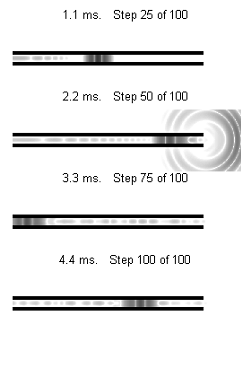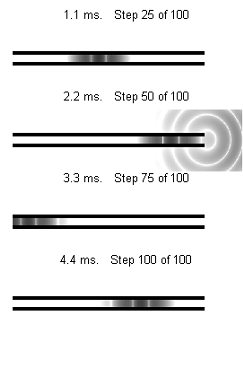Figure 3Relative sound pressure level for Ricker wavelets excitation with central frequency: a) 8 kHz, b) 4 kHz, at different time steps

# 3. RESULTS

Musical instruments which have no flare at the open end of their air column include organ pipes and flutes. For such cases, the sound emitted by these instruments is determined by the characteristics at the open end of the cylindrical pipe. Figure 3 shows sound pressure level at different time steps. (Animations corresponding to that plots can be download at http://ttt.gan.upv.es/~fredondo/).

For the sake of brevity we only present here the results for the reflection function of the termination, i.e, the relationship between the wave propagating backwards and the wave propagating forward. In the frequency domain these two waves are related by the following equation(20)

where r(f), is the reflection function. Figure 4 shows the results obtained for the reflectance of a flanged pipe.Figure 4Reflection coefficient of the termination of the instrument

These results are in good agreement with the theoretical model proposed by Levine and Schwinger  in ,  in which they obtained a rigorous and explicit solution of the reflectance taking into account for the radiation of sound from a circular pipe.

# 4. CONCLUSION

In this paper it is demonstrated that FDTD methods are useful for simulating the acoustic behaviour of a tube, as a first approximation, to analyze the transient characteristics of a wind instrument. In future works we will include in our simulations several terminations of the tube to analyze its effect on the sound radiated by the instrument.

# ACKNOWLEDGEMENT

This work has been supported by the “Ministerio de Ciencia y Tecnología” (CICYT Mat2003-04068).

REFERENCES

     J. Lovetri, D. Mardare and G. Soulodre, Modeling of the seat dip effect using the finite-difference time-domain method, J. Acoust. Soc. Am., 100, 2204 (1996).

     T. Van Renterghem, D. Botteldooren Numerical Simulation of the Effect of Trees on Downwind Noise Barrier Performance. Acta Acustica united with Acustica. Vol. 89 (2003) 764 - 778

       J. O. Smith, Physical modeling using digital waveguides. Computer Music J., vol. 16, no. 4, winter, 74-87.

     J. Martínez, J. Agullo, S. Cardona., Conical bores. Part II: Multiconvolution, J. Acoust. Soc. Am. 84, 1620–1627, 1988

##      M. Walstijn and Murray Campbell. Time-Domain Simulation of Reed Woodwinds with Application to Musical Sound Synthesis. Forum Acusticum meeting, Sevilla 2002.

     K.S. Yee, Numerical solution of initial boundary value problems ivolving Maxwell’s equations in isotropic media;. IEEE Transactions on Antennas Propag., Vol 14, 302-307, 1966

     X. Yuan, D. Borup, J. W. Wiskin, M,. Berggren, R. Eidens, S. Johnson, Formulation and Validation of Berenger’s PML Absorbing Boundary for the FDTD Simulation of Acoustic Scattering;. IEEE Transactions on Ultrasonics, Ferroelectrics, and Frequency control, Vol 44, No. 4, 816-822, July 1997

     X. Yuan, D. Borup, J. W. Wiskin, M,. Berggren, S.A. Johnson, Simulation of acoustic wave propagation in dispersive media with relaxation losses by using FDTD method with PML absorbing Boundary Condition, IEEE Transactions on Ultrasonics, Ferroelectrics, and Frequency control, Vol 46, No. 1, 14-23, January 1999

     N. Ricker, Wavelet contraction, wavelet expansion, and the control of seismic resolution, Geophysics, 18, 769-792. 1953

   J.B. Schneider, C.L. Wagner, S.L. Broschat, Implementation of transparent sources embedded in acoustic finite-difference time domain grids, J. Acoust. Soc. Am. Vol 133, No. 1, 136-142, January 1998

   H. Levine and J. Schwinger, On the radiation of sound from an unflanged circular pipe. Phys. Rev., 73(4):383-406, 1948